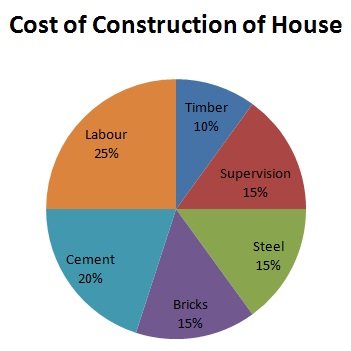# Pie Charts - Solved Examples

Directions(Q 1 to Q 4): The pie-graph given below shows the breakup of the cost of construction of a house. Assuming that the total cost of construction is Rs 600000, answer the question given below:Explanation

```Amount spent on cement= Rs (72/360*600000) = Rs 120000
```

Explanation

```Amount spent on labor=Rs (90/360*600000)=Rs 150000.
Sum spent on steel =Rs (54/360*600000) =Rs 90000
Excess=Rs (150000-90000=Rs 60000
Let 60000=x% of 600000. At that point x/100*600000=60000.
∴ x=10% of aggregate expense.
```

Q 3 - The sum spent on cement, steel and supervision is the thing that percent of the aggregate expense of development?

A - 40%

B - 45%

C - half

D - 55%

Explanation

```Amount spent on concrete, steel and supervision
=Rs {72+54+54/360*600000} =Rs 300000
= half of aggregate expense of development.
```

Q 4 - The sum spent on work surpasses the sum spent on supervision by:

A - Rs 200000

B - Rs 160000

C - Rs 120000

D - Rs 60000

Explanation

```(Amount spent on work)- (Amount spent on supervision)
=Rs (90/360*600000) - Rs (54/360*6000000)
=Rs (150000-90000) =Rs 60000.
```

Directions(Q 5 to Q 9): Study the following information to answer the question given below:Q 5 - What is the distinction in direct enlists and promotee Assistants?

A - 210

B - 280

C - 180

D - None of these

Explanation

```Total number of assistant= 15% of 7000 = (15/100*7000) =1050.
Number of direct recruits= 40% of 1050= (40/100*1050) =420
Number of promotee associates = (1050-420) =630.
Required contrast = (630-420) =210
```

Q 6 - The promotee representative - I is roughly what percent of that of that of direct enlist clerk - I?

A - 10%

B - 9%

C - 10.8%

D - 10.5%

Explanation

```) Number of clerk I =19% of 7000= (7000*19/100) =1330.
Number of direct selects =90% of 1330= (90/100*1330) =1197.
Number of promotes= (1330-1197) =133
∴ required % = (133/1197*100) %= 13300/1197%=10.8%
```

Q 7 - What numbers of workers are supervisors?

A - 1190

B - 1019

C - 1109

D - 1290

Explanation

```Number of supervisors=17% of 7000=(7000*17/100)=1190.
```

Q 8 - What number of aggregate direct enrolls among a wide range of workers arrive?

A - 4000

B - 3885

C - 3000

D - 3115

Explanation

```Average rate of direct selects = 1/3 (30+40+50+90+30+90+70)=400/7%.
Required total=400/7% of 7000= (7000*400/7*1/100) =4000
```

Q 9 - Which sort of workers has most extreme number of direct enrolls?

A - Clerk I & Officer I

B - Officer I

C - Clerk I

D - Clerk II

Explanation

```Total rate of clerk I and officer I= (19+8)%=27%.
Rate of direct Recruits=90% each.
Hence, clerk I and Officer I together have most extreme number of direct enrolls.
```
aptitude_pie_charts.htm